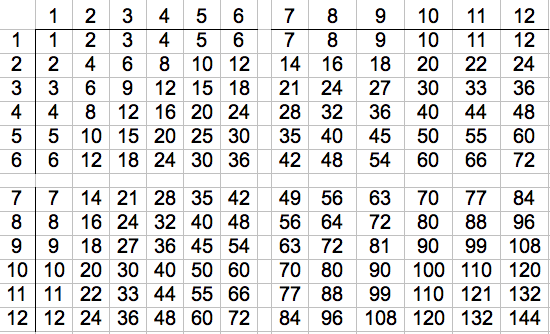# Is There Any Tricks To Learn The Multiplication Facts?

Study them.

Learn the 2-facts and 3-facts.

You already know the 2-facts through 6 from playing dice games, and you know 2*10 from your experience with money. That leaves 7*2, 8*2, and 9*2 to learn.

I learned the 3 facts by learning to count by 3s. 3, 6, 9, 12, 15, 18, 21, 24, 27, 30.

The "4-facts" are double the "2-facts". The "8-facts" are double the "4-facts". The odd 5 facts all end in 5; the even ones all end in 0. The "6-facts" are double the "3-facts".

The "9-facts" are especially interesting. For a given one-digit multiplier, subtract 1 to find the 10s digit. The 1s digit is the value that will make the total be 9.

4*9 = (4-1)_ = 3_ = 36

Given the above, the only ones you have to learn are the 7-facts. You already have most of those because you have the 2, 3, 4, 5, 6, 8, and 9-facts. You're almost done before you start!

I find it easiest to start studying with them in a table on one page. (See
picture) That can make it easier to see the relationships than if you
go at it with flash cards, where you only see them one at a time. (Flash cards can help you practice after you already have learned them.)thanked the writer.